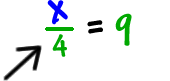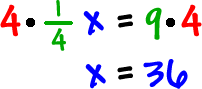Here's another one:

 SolveThere are a couple different ways to deal with this one:

WAY 1:

Rewrite it like this:Since the 4 is dividing into the x,
we'll multiply both sides by
4 to undo him:WAY 2:

Use the fraction fact that 4 is the multiplicative inverse (big word time!) of 1/4:Remember: